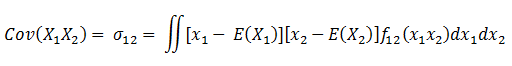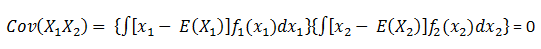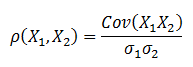# Calculate and Interpret Covariance and Correlations

Covariance defined

In probability theory and statistics, covariance measures the comovement between two variables i.e. the amount by which the two random variables show movement or change together.

If the two variables are dependent then the covariance can be measured using the following formula:For two independent variables the joint densities are separated and the equation becomes:There is a difference between the covariance of two random variables which is linked to the joint probability distribution and the sample covariance which is an estimated value of the parameter.

Covariance interpreted

In financial markets covariance is positive when the variables show similar behaviour i.e. larger values of one variable correspond to larger values of another variable and the same holds true for smaller values.  When the covariance is negative it means the exact opposite i.e. larger values of one variable correspond to smaller values of another variable.

The strength of the linear relationship however cannot be easily interpreted by the magnitude of the calculated value.  In order to interpret the strength a related measure called correlation is used.

Correlation defined

The covariance measure is scaled to a unitless number called the correlation coefficient which in probability is a measure of dependence between two variables.  Dependence broadly refers to any statistical relationship between two variables or two sets of data.

The formula for correlation between two variables is as follows:The covarince is scaled by the product of the two standard devations of the variables. This measure is called the Pearson correlation which holds true only when the relationship between two variables is linear in nature. When the relationship is non-linear in nature Spearman correlation or rank correlation is used in order to account for the deviation from linearity.

Correlation interpreted

Pearson: The correlation number would always be in the range of -1 to +1. A value of 1 means that the variables always move in the same direction and a value of -1 means the two always move in the opposite direction.  In the case where the variables are independent the covariance is zero which means the correlation is also zero. In other words the two variables do not exhibit any movement relative to each other. Any number in between indicates that the one number moves less positively or negatively in relation to changes in another number.

Spearman: This measure is useful when there might be errors in the data and is less sensitive to outliers and more robust.

Refer to the spreadsheet Covariance-Correlation.xlsx for detailed calculations.

# R Programming Bundle: 25% OFF

Get our R Programming - Data Science for Finance Bundle for just $29$39.
Get it now for just \$29